# RD Sharma Solutions For Class 12 Maths Exercise 19.6 Chapter 19 Indefinite Integrals

RD Sharma Solutions for Class 12 Maths Exercise 19.6 Chapter 19 Indefinite Integrals is provided here. Students who aim to top their exams are advised to practice the solutions on a daily basis by referring to RD Sharma Class 12 Solutions. Practising the textbook questions will help you in analyzing your level of preparation and knowledge of the concept.

The pdf of RD Sharma Class 12 Maths can be easily downloaded from the links provided below. This exercise deals with evaluation of integrals of the form

$$\begin{array}{l}\int \sin^{m} x dx, \int \cos ^{m} x dx\end{array}$$
in terms of sines and cosines of multiples of x by using trigonometrical identities.

## Download the PDF of RD Sharma Solutions For Class 12 Chapter 19 – Indefinite Integrals Exercise 19.6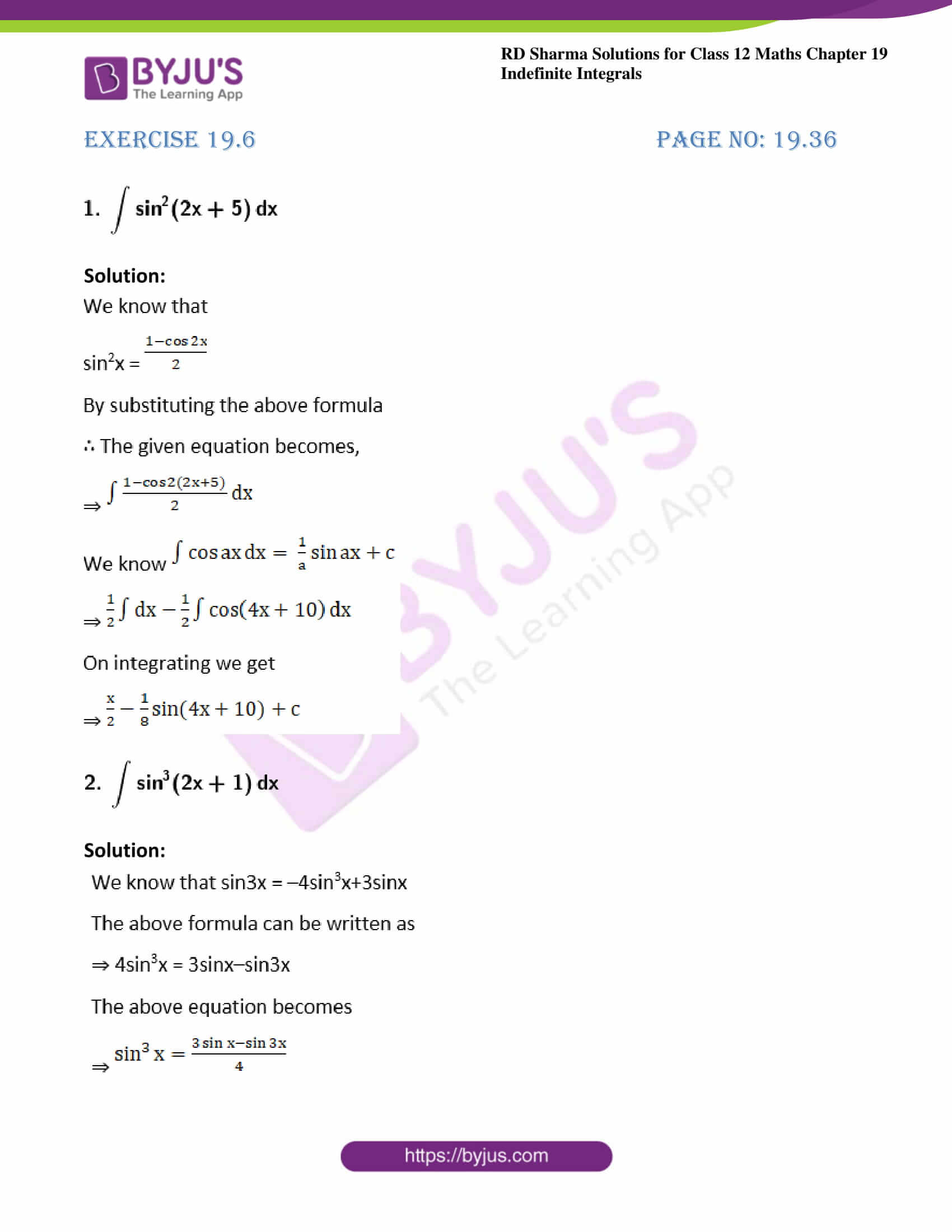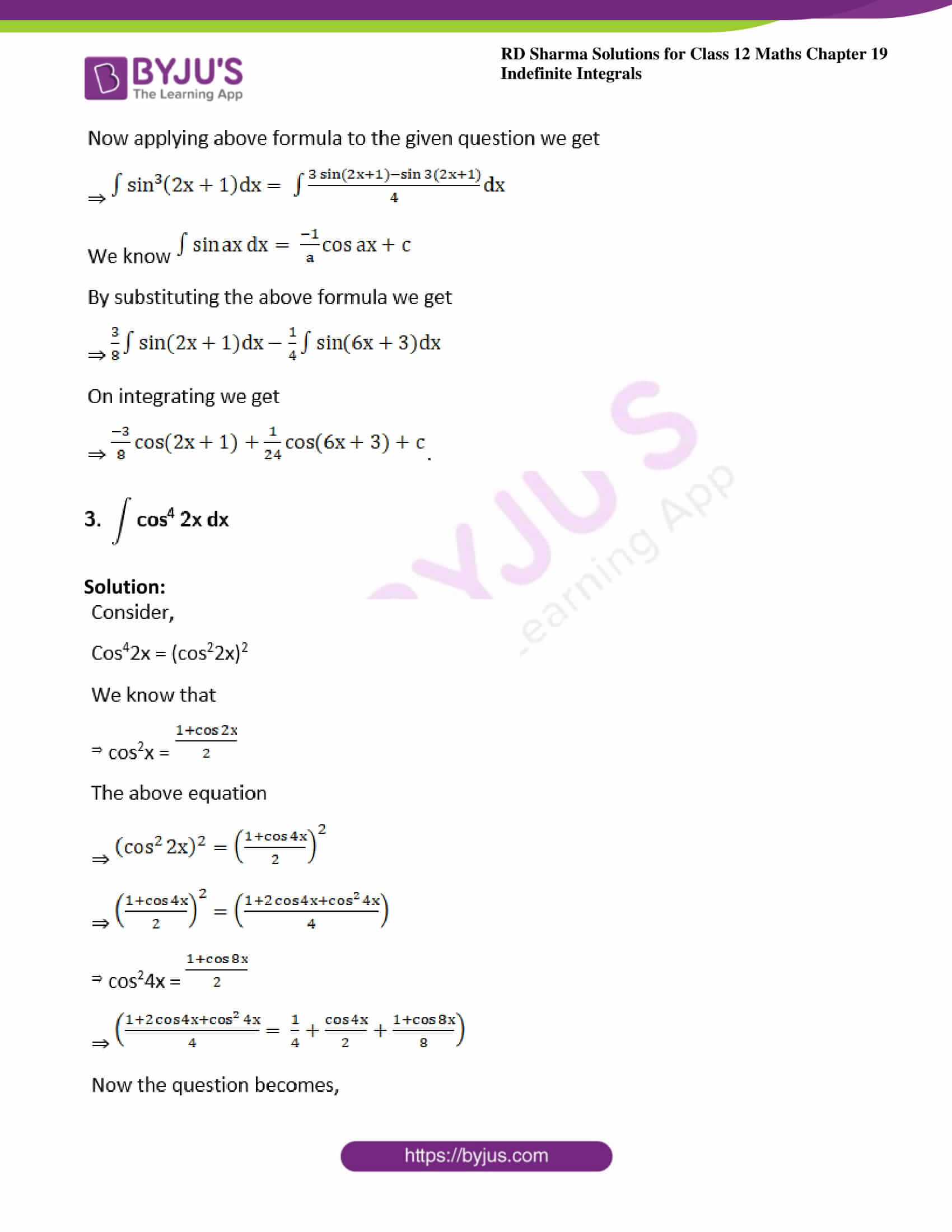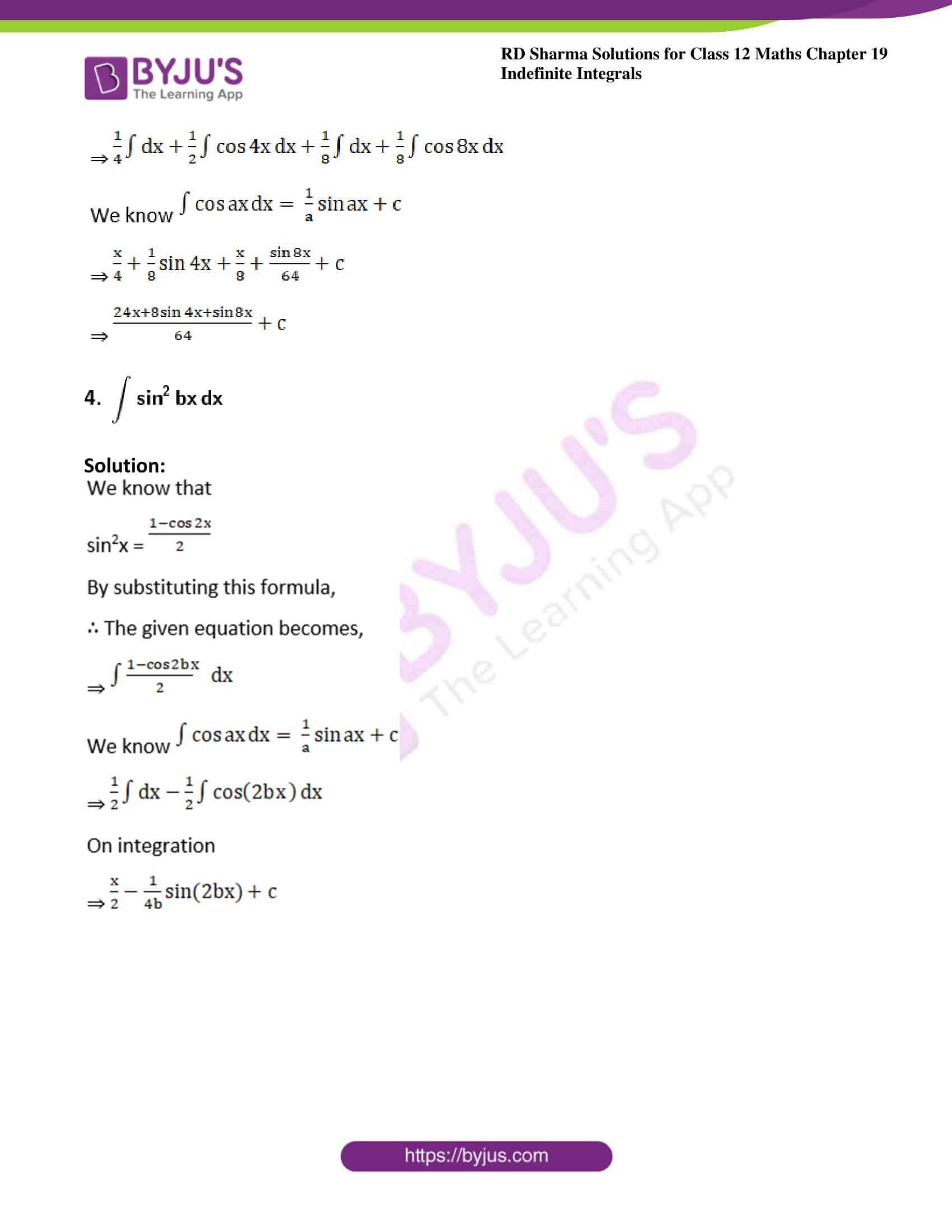### Exercise 19.6 Page No: 19.36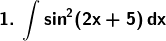Solution: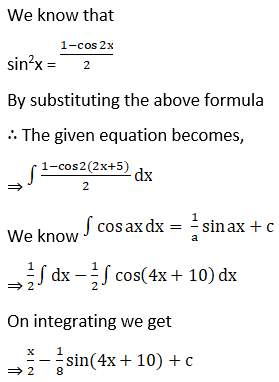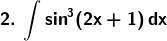Solution: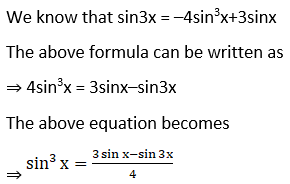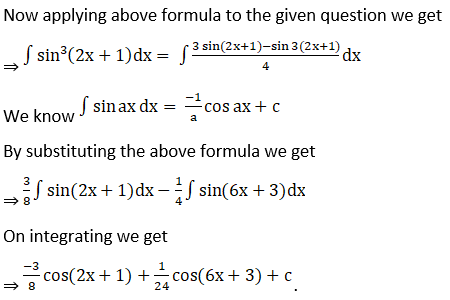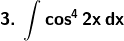Solution: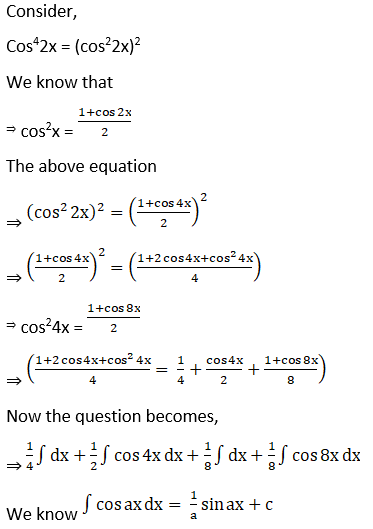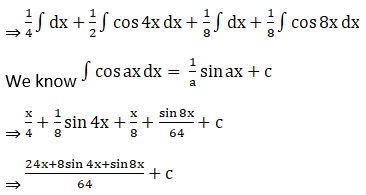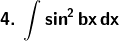Solution: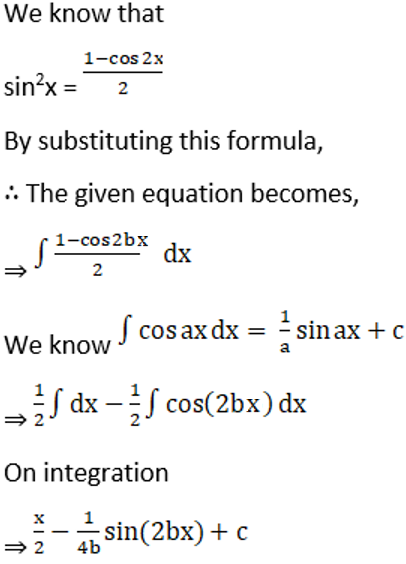### Access other exercises of RD Sharma Solutions For Class 12 Chapter 19 – Indefinite Integrals

Exercise 19.1 Solutions

Exercise 19.2 Solutions

Exercise 19.3 Solutions

Exercise 19.4 Solutions

Exercise 19.5 Solutions

Exercise 19.7 Solutions

Exercise 19.8 Solutions

Exercise 19.9 Solutions

Exercise 19.10 Solutions

Exercise 19.11 Solutions

Exercise 19.12 Solutions

Exercise 19.13 Solutions

Exercise 19.14 Solutions

Exercise 19.15 Solutions

Exercise 19.16 Solutions

Exercise 19.17 Solutions

Exercise 19.18 Solutions

Exercise 19.19 Solutions

Exercise 19.20 Solutions

Exercise 19.21 Solutions

Exercise 19.22 Solutions

Exercise 19.23 Solutions

Exercise 19.24 Solutions

Exercise 19.25 Solutions

Exercise 19.26 Solutions

Exercise 19.27 Solutions

Exercise 19.28 Solutions

Exercise 19.29 Solutions

Exercise 19.30 Solutions

Exercise 19.31 Solutions

Exercise 19.32 Solutions